Next: Cutsets Up: Order vs. Algebraic Topology Previous: (Integer) Homology

## Topological Considerations

Theorem 4.25 can be viewed as a homological analogue of Theorem 3.4. Since the assumptions only require that the retraction is a weak retraction, it is now natural to look for conditions that ensure the existence of such a weak retraction. Most of the work in that direction has been done by Constantin and Fournier in . The connection to topology is established through the topological realization of a simplicial complex: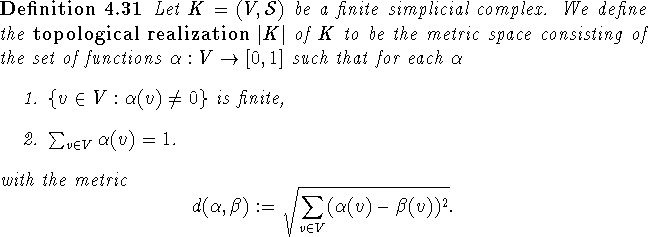Clearly the topological realization of a finite simplicial complex can be embedded as a subspace in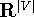with the usual topology. Simplicial maps are extended to affine maps by affine interpolation.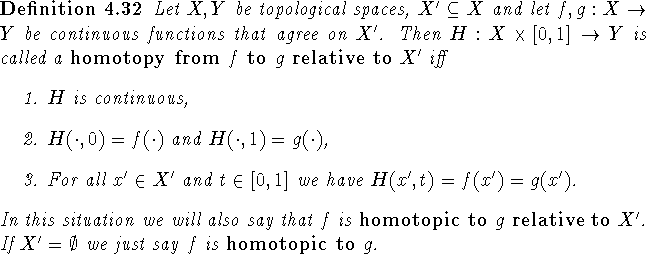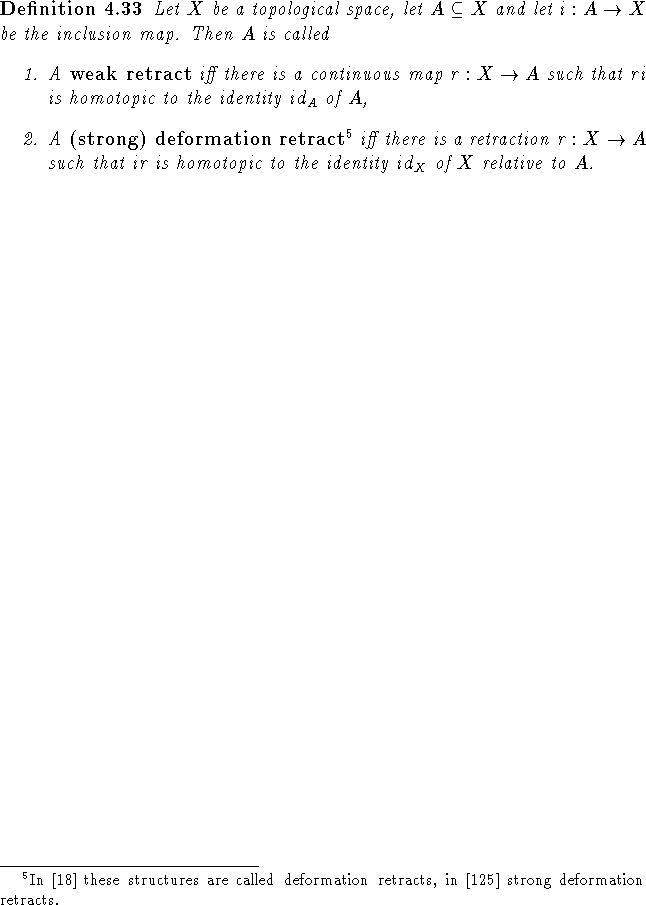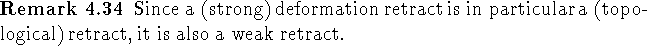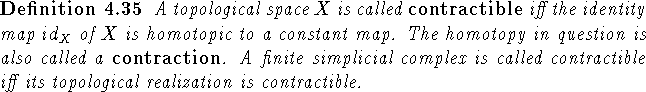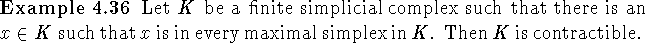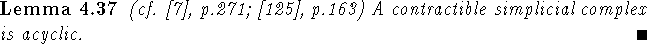From Lemmas 4.22 and 4.24 we can easily infer that if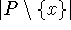is a weak retract of |P| and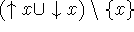is acyclic, then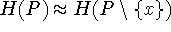. This can be seen as an underlying fact to the work in  in which contractibility (through Proposition 4.39) plays a central role.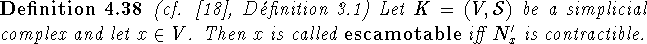Proof: The homotopy of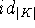to a retraction onto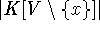relative tois constructed as follows: Let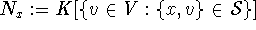and let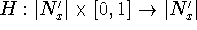be a contraction. Then for each point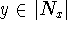there are unique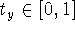and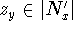such that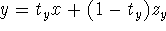and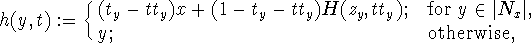is a (strong) deformation retraction from |K| to. \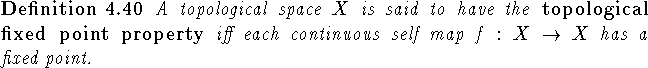For any finite ordered set all the properties below make sense. They are listed such that the lower-numbered properties imply the higher-numbered ones. Similar lists can be made up for graphs and simplicial complexes.

1.   P is dismantlable,
2.   P is ``dismantlable via removing escamotable points",
3. P is contractible,
4.   P is acyclic,
5. |P| has the topological fixed point property,
6. K(P) has the fixed simplex property,
7.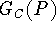has the fixed clique property,
8. P has the fixed point property.

``P is connectedly collapsible" fits in between conditions 1 and 2 (cf. ).Next: Cutsets Up: Order vs. Algebraic Topology Previous: (Integer) Homology

Bernd.S.W.Schroder